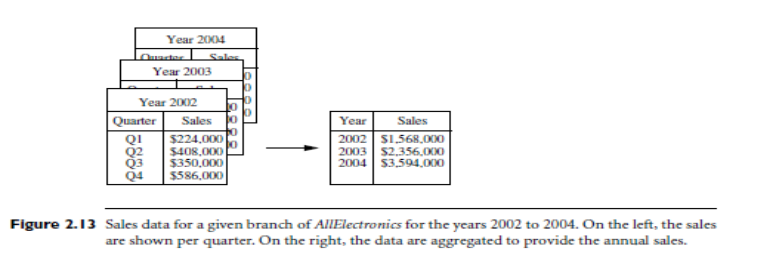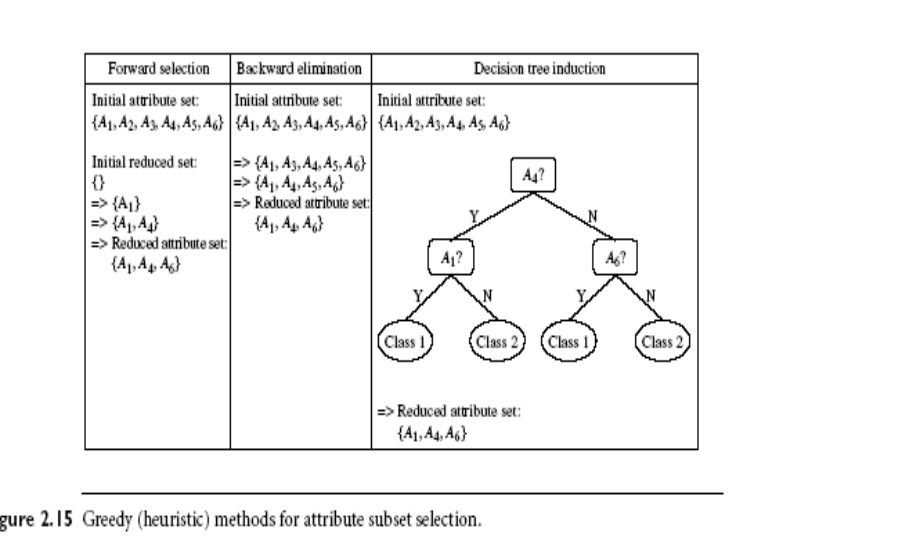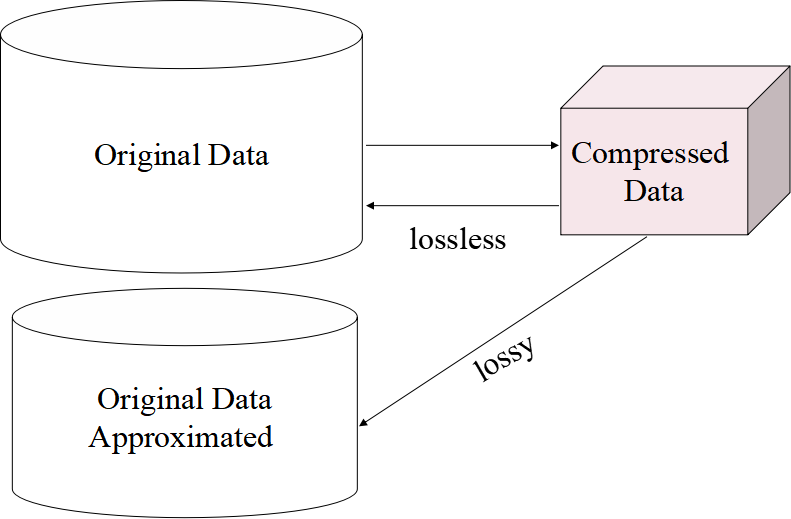# Data Reduction In Data Mining - Various Techniques

## Data Reduction Process

Data Reduction is nothing but obtaining a reduced representation of the data set that is much smaller in volume but yet produces the same (or almost the same) analytical results.

## Data Reduction Methods

• Data cube aggregation
• Dimensionality reduction: e.g., remove unimportant attributes
• Data compression
• Numerosity reduction: e.g., fit data into models
• Discretization and concept hierarchy generation

## Data cube or Dimensional Reduction

Let's consider the situation of a company's data. This data consist of the AllElectronics sales per quarter, for the years 2002 to 2004.

Now we are, generally, interested in the annual sales (total per year), rather than the total per quarter.

Thus the data can be reduced so that the resulting data summarizes the total sales per year instead of per quarter.Data cubes store multidimensional aggregated(summarized) information.
Each cell holds an aggregate data value, corresponding to the data point in multidimensional space.

Data cubes provide fast access to precomputed, summarized data, thereby benefiting on-line analytical processing as well as data mining.

The lowest level of a data cube (base cuboid)
• The cube created at the lowest level of abstraction is referred to as the base cuboid.
• The aggregated data for an individual entity of interest
• E.g., a customer in a phone calling data warehouse
A cube at the highest level of abstraction is the apex cuboid.

Multiple levels of aggregation in data cubes
• Further, reduce the size of data to deal with
Queries regarding aggregated information should be answered using data cube, when possible

## Dimensionality Reduction

### Feature Selection (Attribute Subset Selection):

• Select a minimum set of features such that the probability distribution of different classes given the values for those features is as close as possible to the original distribution given the values of all features
• reduce # of attributes in the discovered patterns, easier to understand

### Heuristic methods (due to exponential # of choices):

• Step-wise forward selection method
• Step-wise backward elimination method
• Combining forward selection and backward elimination method
• Decision-tree induction method

To find Good Subset for Original Attributes
• Let's consider for n attributes, there are 2n possible subsets.
• An exhaustive search for the optimal subset of attributes can be prohibitively expensive, especially as n and the number of data classes increase.
• Therefore, heuristic methods that explore a reduced search space are commonly used for attribute subset selection.
• These methods are typically greedy in that while searching through attribute space, they always make what looks to be the best choice at the time.
• Their strategy is to make a locally optimal choice in the hope that this will lead to a globally optimal solution.
• Such greedy methods are effective in practice and may come close to estimating an optimal solution.
• The “best” (and “worst”) attributes are typically determined using tests of statistical significance, which assume that the attributes are independent of one another.

## Heuristic Feature Selection Methods

1. Best step-wise forward selection:
• Here, the best single-feature is picked first.
• Then the next best feature condition to the first.

2. Step-wise backward elimination:
• In this method, it repeatedly eliminates the worst feature.

3. Best combined forward selection and backward elimination.

4. Optimal branch and bound:
• Use feature elimination and backtracking## Data Compression

1.String compression
• There are extensive theories and well-tuned algorithms
• Typically lossless
• But only limited manipulation is possible without expansion

2. Audio/video compression
• Typically lossy compression, with progressive refinement
• Sometimes small fragments of the signal can be reconstructed without reconstructing the whole

3. The time sequence which is no audio
• Typically short and vary slowly with timeData Compression Diagram

## Numerosity Reduction

1. Reduce data volume by choosing an alternative, smaller forms of data representation

2. Parametric methods
• Assume the data fits some model, estimate model parameters, store only the parameters, and discard the data (except possible outliers)
• Example: Log-linear models -> obtain the value at a point in m-D space as the product on appropriate marginal subspaces

3. Non-Parametric Methods
• Do not assume models
• Major families: histograms, clustering, sampling

### Parametric Methods

Linear regression: Y = w X + b
• Two regression coefficients, w, and b specify the line and are to be estimated by using the data at hand
• Using the least-squares criterion to the known values of Y1, Y2, …, X1, X2, ….
Multiple regression: Y = b0 + b1 X1 + b2 X2.
• Many nonlinear functions can be transformed into the above
Log-linear models:
• The multi-way table of joint probabilities is approximated by a product of lower-order tables
• Probability:  p(a, b, c, d) = ab acad bcd

## Discretization & Concept Hierarchy

1. Discretization
• Discretization is the method which reduces the number of values for a given continuous attribute by dividing the range of the attribute into intervals
• For this interval, labels can then be used to replace actual data values
• Supervised vs. unsupervised
If the Discretization process used class information then we say supervised.
• Split (top-down) vs. merge (bottom-up)
• Discretization can be performed recursively on an attribute

2. Concept hierarchy formation
• Recursively reduce the data by collecting and replacing low-level concepts (such as numeric values for age) by higher-level concepts (such as young, middle-aged, or senior)

Typical methods: All the methods can be applied recursively
1. Binning
• Top-down split, unsupervised.
2. Histogram analysis
• Top-down split, unsupervised.
3. Clustering analysis (covered above)
• Either top-down split or bottom-up merge, unsupervised.
4. Entropy-based discretization: supervised, top-down split.

5. Interval merging by chi^2 Analysis: unsupervised, bottom-up merge.

6. Segmentation by natural partitioning: top-down split, unsupervised.
(We will be discussing those separately each topic in a separate article).